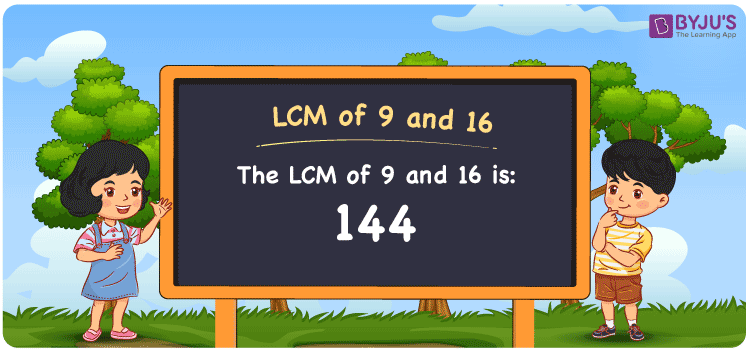Checkout JEE MAINS 2022 Question Paper Analysis : Checkout JEE MAINS 2022 Question Paper Analysis :

# LCM of 9 and 16

LCM of 9 and 16 is 144. The smallest number among all common multiples of 9 and 16 is 144. We can determine the LCM of 9 and 16 with the help of prime factorisation, division method and listing multiples. (9, 18, 27, 36, 45, 54, 63, ….) and (16, 32, 48, 64, 80, 96….) are the first few multiples of 9 and 16. Students can make use of the article HCF and LCM to learn tricks of finding LCM and HCF more effectively. Let us grasp the technique of finding the least common multiple of 9 and 16 with examples and FAQs in a comprehensive manner in this article.

## What is LCM of 9 and 16?

The Least Common Multiple of 9 and 16 is 144.## How to Find LCM of 9 and 16?

The following methods help us to find the LCM of 9 and 16:

• Prime Factorisation
• Division method
• Listing the multiples

### LCM of 9 and 16 Using Prime Factorisation Method

By prime factorisation method, we can write 9 and 16 as the product of prime numbers, such that;

9 = 3 × 3

16 = 2 × 2 × 2 × 2

LCM (9, 16) = 2 × 2 × 2 × 2 × 3 × 3 = 144

### LCM of 9 and 16 Using Division Method

Here, we divide the numbers 9 and 16 by their prime factors to find their LCM. The product of these divisors represents the least common multiple of 9 and 16.

 2 9 16 2 9 8 2 9 4 2 9 2 3 9 1 3 3 1 x 1 1

No more further division can be done.

Therefore, LCM (9, 16) = 2 × 2 × 2 × 2 × 3 × 3 = 144

### LCM of 9 and 16 Using Listing the Multiples

To determine the least common multiple of 9 and 16 using listing multiples, we list out the multiples of 9 and 16 as shown below.

Multiples of 9: 9, 18, 27, 36, 45, 54, 63, 72, 81, 90, ……., 126, 135, 144, ……..

Multiples of 16: 16, 32, 48, 64, 80, 96, 112, 128, 144, ……..

LCM (9, 16) = 144

## Related Articles

Least Common Multiple (LCM)

LCM of Two Numbers

Prime Factorization and Division Method for LCM and HCF

LCM Formula

LCM with Examples

LCM Calculator

## Video Lesson on Applications of LCM## Solved Examples

Q. 1: Find the LCM of 9 and 16 using listing multiples?

Solution: First, list the multiples of 9 and 16

Multiples of 9 = 9, 18, 27, 36, 45, 54, 63, 72, 81, 90, 99, 108, 117, 126, 135, 144, 153, …..

Multiples of 16 = 16, 32, 48, 64, 80, 96, 112, 128, 144, 160, ……..

Here, the LCM is 144

Hence the LCM of 9 and 16 is 144.

Q. 2: The product of two numbers is 144. If their GCD is 1, calculate their LCM.

Solution: Given

GCD = 1

Product of numbers = 144

We know that,

LCM × GCD = Product of numbers

LCM = Product of numbers/GCD

LCM = 144/1

LCM = 144

Thus the LCM is 144.

## Frequently Asked Questions on LCM of 9 and 16

### What is the LCM of 9 and 16?

The LCM of 9 and 16 is 144.

### How do you find the LCM of 9 and 16 by Prime Factorisation?

9 = 3 × 3

16 = 2 × 2 × 2 × 2

LCM (9, 16) = 2 × 2 × 2 × 2 × 3 × 3 = 144

Hence the LCM of 9 and 16 is 144.

### Name the methods used to determine the LCM of 9 and 16.

The following methods are used to determine the LCM of 9 and 16

Prime Factorisation Method

Division Method

Listing the Multiples